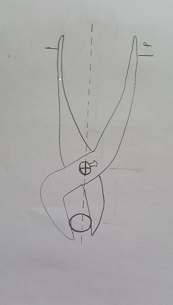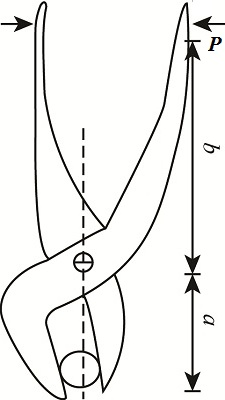# The Bolt B is held by a gripping force of 8.5 lb perpendicular to the jaws of the pliers as shown...

## Question:

The Bolt {eq}B {/eq} is held by a gripping force of {eq}8.5 lb {/eq} perpendicular to the jaws of the pliers as shown in the figure below. What forces {eq}P{/eq} must be applied perpendicular to the handles to supply the gripping force?## Moment

The term moment is defined as a measure that is able to start rotation in a body when applied on it. The moment is also termed as torque. The moment is determined as a product of the force and the position at which the force is applied.

## Answer and Explanation:

Given Data:

• The gripping force required to hold the bolt is: {eq}{F_{bg}} = 8.5\;{\rm{lb}} {/eq}
• The distance between the blot and hinge joint is: {eq}{L_{ja}} = a {/eq}
• The distance between the joint and hand grip is: {eq}{L_{jb}} = b {/eq}

Free Body Diagram of pliersThe expression for the moment at hinge joint,

{eq}{F_{bg}} \times {L_{ja}} - P \times {L_{jb}} = 0 {/eq}

Here P is the force required to hold the bolt.

Substitute the given data in above expression,

{eq}\begin{align*} 8.5 \times a &= P \times b\\ P &= \dfrac{{8.5a}}{b}\;{\rm{lb}} \end{align*} {/eq}

Thus the force required to hold the bolt is {eq}\dfrac{{8.5a}}{b}\;{\rm{lb}} {/eq}

#### Learn more about this topic:Torque in Physics: Equation, Examples & Problems

from Physics: Middle School

Chapter 3 / Lesson 13
43K